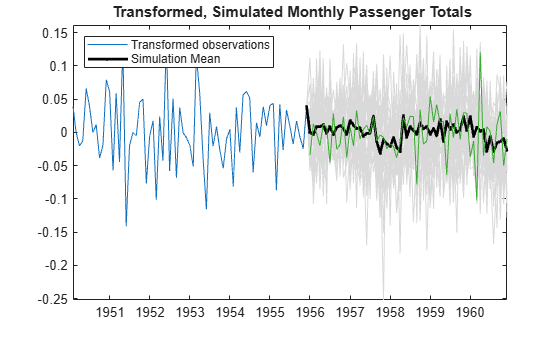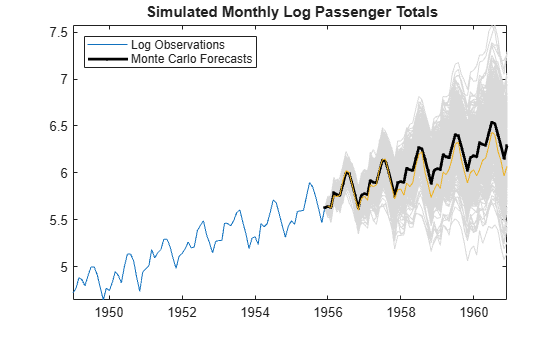## Simulate Regression Models with Multiplicative Seasonal Errors

### Simulate a Regression Model with Stationary Multiplicative Seasonal Errors

This example shows how to simulate sample paths from a regression model with multiplicative seasonal ARIMA errors using simulate. The time series is monthly international airline passenger numbers from 1949 to 1960.

Load the airline and recessions data sets.

load('Data_Airline.mat') load Data_Recessions

Transform the airline data by applying the logarithm, and the 1st and 12th differences.

y = Data; logY = log(y); DiffPoly = LagOp([1 -1]); SDiffPoly = LagOp([1 -1],'Lags',[0, 12]); dLogY = filter(DiffPoly*SDiffPoly,logY);

Construct the predictor (X), which determines whether the country was in a recession during the sampled period. A 0 in row t means the country was not in a recession in month t, and a 1 in row t means that it was in a recession in month t.

X = zeros(numel(dates),1); % Preallocation for j = 1:size(Recessions,1) X(dates >= Recessions(j,1) & dates <= Recessions(j,2)) = 1; end X = X(14:end); % Remove the first 14 observations for consistency dates = dates(14:end);

Define index sets that partition the data into estimation and forecast samples.

nSim = 60; % Forecast period T = length(dLogY); estInds = 1:(T-nSim); foreInds = (T-nSim+1):T;

Estimate the regression model with multiplicative seasonal errors:

${y}_{t}=c+{X}_{t}\beta +{u}_{t}$

${u}_{t}=\left(1+BL\right)\left(1+{B}_{12}{L}^{12}\right){\epsilon }_{t}.$

Mdl = regARIMA('MALags',1,'SMALags',12); EstMdl = estimate(Mdl,dLogY(estInds),'X',X(estInds));
 Regression with ARMA(0,1) Error Model with Seasonal MA(12) (Gaussian Distribution): Value StandardError TStatistic PValue _________ _____________ __________ __________ Intercept 0.0042146 0.0015333 2.7486 0.0059843 MA{1} -0.47713 0.12087 -3.9473 7.9044e-05 SMA{12} -0.74115 0.12042 -6.1549 7.5116e-10 Beta(1) -0.018912 0.0075665 -2.4994 0.012439 Variance 0.0016695 0.00031169 5.3564 8.4901e-08 

Use the estimated coefficients of the model (contained in EstMdl) to simulate 25 realizations of airline passenger counts over the 60-month horizon. Infer the residuals, and use them as a presample.

[~,u0] = infer(EstMdl,dLogY(estInds),'X',X(estInds)); rng(5); numPaths = 25; dLogYSim = simulate(EstMdl,60,'numPaths',numPaths,'U0',u0,'X',X(foreInds)); meanDLogYSim = mean(dLogYSim,2); figure h1 = plot(dates(estInds),dLogY(estInds)); title('{\bf Transformed, Simulated Monthly Passenger Totals}') hold on plot(dates(foreInds),dLogYSim,'Color',[.85,.85,.85]) h2 = plot(dates(foreInds),meanDLogYSim,'k.-','LineWidth',2); plot([dates(estInds(end)),dates(foreInds(1))],... [repmat(dLogY(estInds(end)),numPaths,1),dLogYSim(1,:)'],... 'Color',[.85,.85,.85]) plot([dates(estInds(end)),dates(foreInds(1))],... [dLogY(estInds(end)),meanDLogYSim(1)],'k.-','LineWidth',2) plot(dates(foreInds),dLogY(foreInds)) datetick legend([h1,h2],'Observations','Simulation Mean','Location','NorthWest') axis tight hold offThe regression model with SMA errors seems to forecast the series well.

Check the predictive performance of the model by:

1. Varying the size of the forecast period

2. Estimating the prediction mean square error (PMSE)

3. Choosing the model with the lowest PMSE

### Untitled

Simulate a Regression Model with Nonstationary Multiplicative Seasonal Errors

This example shows how to simulate sample paths from a regression model with multiplicative seasonal ARIMA errors using simulate. The time series is monthly international airline passenger numbers from 1949 to 1960.

Load the airline and recessions data sets. Transform the response.

load('Data_Airline.mat') load Data_Recessions y = log(Data);

Construct the predictor (X), which determines whether the country was in a recession during the sampled period. A 0 in row t means the country was not in a recession in month t, and a 1 in row t means that it was in a recession in month t.

X = zeros(numel(dates),1); % Preallocation for j = 1:size(Recessions,1) X(dates >= Recessions(j,1) & dates <= Recessions(j,2)) = 1; end

Define index sets that partition the data into estimation and forecast samples.

nSim = 60; % Forecast period T = length(y); estInds = 1:(T-nSim); foreInds = (T-nSim+1):T;

Estimate the regression model with multiplicative seasonal errors:

${y}_{t}={X}_{t}\beta +{u}_{t}$

$\left(1-L\right)\left(1-{L}^{12}\right){u}_{t}=\left(1+BL\right)\left(1+{B}_{12}{L}^{12}\right){\epsilon }_{t}.$

Set the regression model intercept to 0 since it is not identifiable in an integrated model.

Mdl = regARIMA('D',1,'Seasonality',12,'MALags',1,'SMALags',12,... 'Intercept',0); EstMdl = estimate(Mdl,y(estInds),'X',X(estInds));
 Regression with ARIMA(0,1,1) Error Model Seasonally Integrated with Seasonal MA(12) (Gaussian Distribution): Value StandardError TStatistic PValue _________ _____________ __________ __________ Intercept 0 0 NaN NaN MA{1} -0.35662 0.10393 -3.4312 0.00060088 SMA{12} -0.67729 0.11294 -5.9972 2.0077e-09 Beta(1) 0.0015098 0.020533 0.07353 0.94138 Variance 0.0015198 0.00021411 7.0983 1.2631e-12 

Use the estimated coefficients of the model (contained in EstMdl), to simulate airline passenger counts over the 60-month horizon. Infer the residuals, and use them as a presample.

[e0,u0] = infer(EstMdl,y(estInds),'X',X(estInds)); rng(5); numPaths = 500; ySim = simulate(EstMdl,nSim,'numPaths',numPaths,'E0',e0,... 'U0',u0,'X',X(foreInds)); meanYSim = mean(ySim,2); figure h1 = plot(dates(estInds),y(estInds)); title('{\bf Simulated Monthly Passenger Totals}') hold on plot(dates(foreInds),ySim,'Color',[.85,.85,.85]) h2 = plot(dates(foreInds),meanYSim,'k.-','LineWidth',2); plot([dates(estInds(end)),dates(foreInds(1))],... [repmat(y(estInds(end)),numPaths,1),ySim(1,:)'],... 'Color',[.85,.85,.85]) plot([dates(estInds(end)),dates(foreInds(1))],... [y(estInds(end)),meanYSim(1)],'k.-','LineWidth',2) plot(dates(foreInds),y(foreInds)) datetick legend([h1,h2],'Observations','Monte Carlo Forecasts',... 'Location','NorthWest') axis tight hold offThe simulated forecasts show growth and seasonal periodicity similar to the observed series. The regression model with SMA errors seems to forecast the series well, albeit slightly overestimating.

Check the predictive performance of the model by:

1. Varying the size of the forecast period

2. Estimating the prediction mean square error (PMSE)

3. Choosing the model with the lowest PMSE